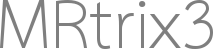# Tractography parameters explicitation

Dear Mrtrix community,

I am starting to use Mrtrix on the HCP data. I would like to perform a FSL like tractography (i.e. exploring the complete signal space given probabilistic a set of tractography parameters) but keeping the geometry of the fibers and the advantages of MSMT model and iFOD2.
I read the tckgen doc but there are some parameters I am not sure to understand properly.

• trials : at each point at certain number of samples are drawn from FOD distribution and each trial is becoming a "new streamlines "?
• power : why powering the FOD by the inverse of the number of samples ?
• max_attempts_per_seed : should I use this param to obtain several fibers from one seed (exploring the FOD distribution)

If you have any hint to helpI’m not sure I understand what you mean here. Which aspect of the FSL tractography pipeline are you trying to replicate? I suspect one of the various seeding options will be suitable for what you’re after (maybe `-seed_grid_per_voxel`?), but hard to know from your statement…

No, this is a parameter used for the rejection sampling that is used to obtain a fresh random sample from the fibre ODF at each step of the streamline. Briefly, at each step, the streamlines algorithm needs to determine the direction of the next step. With deterministic tracking, that’s just the nearest peak in the fODF (of the major eigenvector if using tensor tracking). With probabilistic approaches, we need to draw a random direction from the fODF - in `tckgen`, we use rejection sampling for this. It works by drawing a sample direction from the uniform distribution, then accepting this direction with probability proportional to the amplitude of the fODF. Obviously that means many samples will be rejected before we find one that is accepted. The `-trials` option sets how many attempts to do at each step in the streamline before giving up. The idea being that if the fODF is sub-threshold over the entire acceptable range of directions, then no samples will ever be accepted, so we need to give up at some point and say that the fODF is too small to track at this point, and hence we terminate the streamline.

Because if the probability of a path segment is the product of the probabilities for the path tangent at each sample point, then this path segment probability becomes dependent on the number of sample points. But if we take the product of sample point probabilities and raise it to the power 1/ nsamples, then that should counter that effect (in practice, it’s a little messier than that, we’re still discussing the best way to deal with this internally - but I won’t go into it here).

Ah, OK, I think this answer my first point.

No, this controls how hard to try finding a suitable starting direction from a given seed point. The idea being that the initial direction of tracking should also be selected at random, but most directions will not be eligible due to being sub-threshold. This is the same issue as the `-trials` issue above. If there are no suitable supra-threshold directions in the fODF for that seed point, we need to stop trying to find such a direction at some point. This is what this option does. But once a suitable direction is selected, the algorithm will not re-visit that seed point.

I think what you’re after is probably the `-seed_random_per_voxel` or `-seed_grid_per_voxel` options. These will guarantee the same number of (attempted) seed points for each voxel, although the locations of these seed points will be distributed throughout the voxel, rather than limited to the centre of the voxel.

Thank you very much for this quick and super complete answerI was trying to “explore” the signal so that reconstructed streamlines will be stable from a FOD point of view. To do so I wanted to have enough seeds (either at the gwmi or in the wm) and use probabilistic tracking to sample the whole FOD distribution. The goal is to get “all” possible streamlines from the calculated FOD point of view .

Hi professor tournier，
I want to do the probabilistic tracking,and I want to know how to control the probability of the fibers. For example, when I track the Corticospinal tracts using the probablistic algorithm，I just want the fibers which have a probability of over 80%.Could the option -power make a role of controlling the probability ？
Cheers，
Liang

Hi Liang,

Individual streamlines do not themselves have a “probability”; the term “probabilistic” in the context of streamlines tractography refers only to the presence of some stochastic process in the process of reconstruction (typically in reference to the determination of the orientation in which to propagate the streamline).

Rob